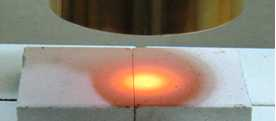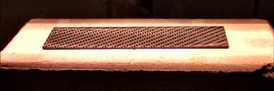# 5-2.Heat balance equation of Infrared ray dryingThe electrical energy (electric power) supplied from the power supply serves as electromagnetic waves, and jumps out of a heating element.
The electromagnetic waves exist wavelength of various kinds, but the most effective in heating is infrared rays.
The low- heat side (heating object) absorbs the electromagnetic waves radiated from the high-fever side (heater).
This is infrared heating.

### 5-2-1.Efficiency of the heater ” He” can be calculated by the equation below.

He = (R + A + T) / P

Rewriting

He = (P - Hl) / P

He: efficiency of the heater
Hl: heat radiation loss from heater
R : reflection rate
A : absorption rate
T : transparent rate
P : power

The “He” halogen heater boasts the best of efficiency in a variety of heater at about 85%.

### 5-2-2.Heating efficiency Oe of the object to be heated can be calculated by the equation below.

Oe = A - Ol

Oe: heating efficiency of the heating object
A : absorption rate
Ol: heat radiation loss from the object to be heated

Infrared absorption rate of each material – please refer to the “Science of the Infrared rays – 6. Absorption rate of infrared rays“.

### 5-2-3.Amount of heat J needed for heating can be calculated by the following formula.

J = Ow x cv x Ot

Ow: the mass of the object to be heated
cv: specific heat at constant volume of the object to be heated
Ot: rising temperature width

Specific heat of each material – please refer to the “Science of the Infrared rays – 10. The main materials Specific gravity,specific heat and thermal conductivity“.

### 5-2-4.Power Ph necessary to infrared heating can be calculated by the following formula.

Ph = J / (He x Oe) / (P x Ar)